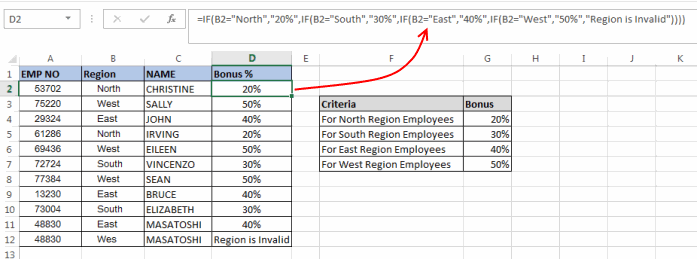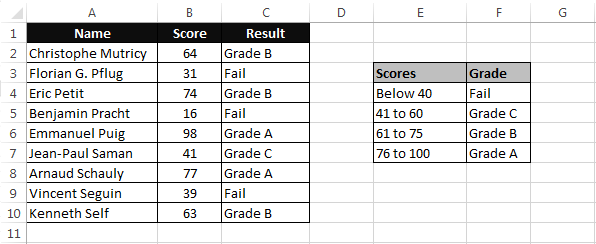# Excel Nested If’s – Explained

In our last post, we talked about the IF Statement, which is one of the most important functions in Excel.  The limitation of the IF statement is that it has only two outcomes. But if you are dealing with multiple conditions then Excel Nested If’s can come in very handy.

Nested if’s are the formulas that are formed by multiple if statements one inside another. This nesting makes it possible for a single formula to take multiple decisions. In Excel 2003 nesting was only possible up to 7 levels but Excel 2007 has increased this number to 64.

## Syntax of Excel Nested IF formula:

The syntax of the nested IF statement is as follows:

`=IF(Condition_1,Value_if_True_1,IF(Condition_2,Value_if_True_2,Value_if_False_2))`

Here, `‘Condition_1’` refers to the condition used in the first IF.
`‘Value_if_True_1’` will be the result if the first IF statement is True.
`‘Condition_2’` is the condition used in the second IF. The second IF will only come into picture when the First IF statement results a False value.
`‘Value_if_True_2’` will be the result if the second IF statement is True.
`‘Value_if_False_2’` will be the result if the second IF statement is False.

This is equivalent to:

`IF Condition1 = true THEN value_if_true1 'If Condition1 is trueELSE IF Condition2 = true THEN value_if_true2 'If Condition2 is trueELSE value_if_false2 'If both conditions are falseEND IF 'End of IF Statement`

## Example of Nested IF’s in Excel:

Now, let’s understand Nested If’s with an example.

Example 1:

In the below image an Employee table of a company is shown. The company decides to give a bonus to its employees but their bonus criteria is quite strange. As you can see in the below image they are giving 20% bonus to the North Region Employees, 30% to the South Region Employees, 40% to the East Region Employees and 50% to the West Region Employees.In this case, we can use Excel Nested IF formula to find the bonus for each employee. The formula can be:

`=IF(B2="North","20%",IF(B2="South","30%",IF(B2="East","40%",IF(B2="West","50%", "Region is Invalid"))))`

The formula is quite simple, it just checks if ‘B2’ (the cell that contains region details for the first employee) is equal to “North”, then the value should be 20%, if not then check if B2 is equal to “South”, if yes then the value should be 30%, if not move on to next IF statement and so on.

Similarly, for the second employee the formula would be:

`=IF(B3="North","20%",IF(B3="South","30%",IF(B3="East","40%",IF(B3="West","50%", "Region is Invalid"))))`

In this case, I have handled another important thing i.e. If the Region does not match with any one of the IF conditions then the output should be “Region is Invalid”.

Example 2:

In the second example, we have a table of students and their scores. Now based on their scores we have to give a grade to the students.Students with scores below 40 are considered as “Fail”, scores between 41 and 60 are considered “Grade C”, scores between 61 and 75 are considered “Grade B” and scores between 76 and 100 are considered as “Grade A”

In this scenario, we can use a nested If formula as:

`=IF(B2<=40,"Fail",IF(AND(B2>=41,B2<=60),"Grade C",IF(AND(B2>=61,B2<=75),"Grade B",IF(AND(B2>=76,B2<=100),"Grade A"))))`

This formula just checks if B2 (cell containing the score of the first student) is less than or equal to 40, if true then the value should be “Fail” if not then check the next IF condition and so on.

You can see that here in the inner-most IF statement I haven’t used the ‘Value_if_False’, it is perfectly alright to omit this parameter in such a case. In-case all the IF conditions in this formula will result in a False value then the formula will simply return a ‘FALSE’ keyword.

So, this was all about Nested IF Instruction. Feel free to drop your comments related to the topic.About Ankit Kaul

Ankit is the founder of Excel Trick. He is tech Geek who loves to sit in front of his square headed girlfriend (his PC) all day long. :D. Ankit has a strong passion for learning Microsoft Excel. His only aim is to turn you guys into 'Excel Geeks'.